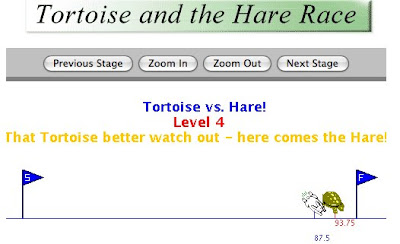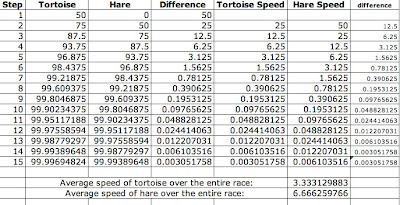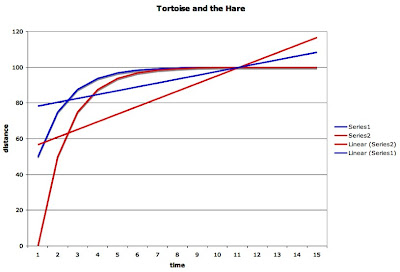## Saturday, January 26, 2008

### Friday Fractal: Lesson 1: Achilles and the TortoiseI want to understand fractals better, and in the hope that somebody else also wants to, I am going to post each lesson that I complete. I have chosen to study the Introduction to Fractals: Infinity, Self-Similarity and Recursion published by Shodor. There's no special reason why I chose this lesson. But because I did, we will reviewing Zeno's Paradox. There are several paradoxes of Zeno but basically they argue that you can't travel anywhere at all because you can divide the distance to an object in half and in half again and again and never quite reach it. That is a very quick and dirty summary of the paradoxes. To learn the details, read the Wikipedia article Zeno's Paradoxes. More on the paradox in this lesson later.

First, we need to define some words. Keep these definitions around because we will be using them for the next five lessons. If there is a link attached to a word, it will take you to a Shodor "discussion" of the topic (in a new window). Since we want to start our learning nice and easy, we are going to read these discussions thoroughly.

Vocabulary
• fractal: a term coined by Benoit Mandelbrot in 1975, referring to objects built using recursion, where some aspect of the limiting object is infinite and another is finite, and where at any iteration, some piece of the object is a scaled down version of the previous iteration
• generator: the bent line-segment or figure that replaces the initiator at each iteration of a fractal.
• infinity: Greater than any fixed counting number, or extending forever. No matter how large a number one thinks of, infinity is larger than it. Infinity has no limits
• initiator: a line-segment or figure that begins as the beginning geometric shape for a fractal. The initiator is then replaced by the generator for the fractal.
• iteration: repeating a set of rules or steps over and over. One step is called an iterate.
• logarithms: the exponent of the power to which a base number must be raised to equal a given number. An example: 2 is the logarithm of 100 to the base 10. One can look at this way: 10 * 10 = 100, which is the same as 10^2, and 2 is the exponent referred to above.
• recursion: given some starting information and a rule for how to use it to get new information, the rule is then repeated using the new information
• self-similarity: two or more objects having the same characteristics. In fractals, the shapes of lines at different iterations look like smaller versions of the earlier shapes
• Properties of Fractals Discussion: Mandelbrot used the word "fractal" because in Latin, fractus means broken. Mandelbrot viewed these things as being highly irregular and crinkley. Another good reason to use the word fractal is that they have fractional dimension. See, also, notes on plane figure fractals discussion below.
• Dimension and Scale Discussion: Dimension, Scale and Number of copies: Scale ^ Dimension = Number of copies.
• Exponents and Logarithms Discussion: most calculators have a button built in to calculate logs. The Koch Snowflake is not one dimensional or two dimensional but somewhere in the middle. On a calculator, E12 on the end says to move the decimal point 12 spaces right. When we use the log base e we call it the Natural Log (ln). I'll need to refer to this discussion a lot.
• Plane Figure Fractals Discussion: things all fractals have in common: (1) All were built by starting with an "initiator" and "iterating" using a "generator." So we used recursion.
(2) Some aspect of the limiting object was infinite (length, perimeter, surface area) — Many of the objects got "crinklier." (3) Some aspect of the limiting object stayed finite or 0 (area, volume, etc). (4) At any iteration, a piece of the object is a scaled down, otherwise identical, copy of the previous iteration (self-similar).
• Infinity and Iteration Discussion: The sum of an infinite number of numbers can be finite. Think about the tortoise and hare race: The tortoise travels the following distances, one fraction for each time step: 1/2 + 1/4 + 1/8 + 1/16 + 1/32 + . . . but never gets to the end of the race! So the sum above never gets past 1. AND So when we ran the tortoise and hare race, each time I press the advance button in the Tortoise and the Hare I am iterating: repeating a rule over and over again. So I must find the rule (it looks like the rule is half your distance each step). AND infinite sums --> calculus --> limits. AND "repeat infinitely many times:" means let the number of iterations approach infinity.
• Recursion Discussion: recursion: a special kind of iteration. It's a rule for moving to the next stage. Fibonacci sequence is generally extended with a recursive rule (add the previous two numbers to get the next term).
• Self-Similarity Discussion: it's a property of the object, not of the steps used to build the object. Iteration and recursion frequently result in self-similar objects. Hilbert Curve is a self-similar object, but the way we construct it is an iterative process.
• Trees As Data Structures Discussion: outcomes, probabilities, binary trees, how to make many dimensional trees, and logarithms! Cool stuff! Keep for the future. It ties all the stuff in school together, now I know why it's all important in my classes.
• Wikipedia: Zeno's Paradoxes (take what you can and leave the rest)I played with the Tortoise and Hare applet and recorded the results so that I could complete the worksheet. I immediately recognized the race as one of Zeno's paradoxes and had a lot of fun with it. Here are my results:Neither runner gets to the finish line. The differences in speed and length run are powers of two. The rule seems to be to cut the distance in half in each time unit. My right-most column in the spreadsheet is not labeled or formatted correctly because it is for only one racer. If you drop the data down one row, the differences are identical for the hare.I knew the graph would be a curve and would approach one. I drew fitted lines for each runner and they intersected somewhere between 11 and 12 or something (I can't see it clearly and didn't take the time to figure it out but it would be easy enough).The graph of the speed of each runner: they approach zero. The runners' fitted lines intersect between 10 and 11.

Worksheet Questions:

Who is ahead after 5, 10 and 15 time steps? By how much? The tortoise is always ahead by 1/2 of the distance he was ahead by before.

Who is running faster? Calculate the average speed of the tortoise and the hare during each of the first four stages. Remember that the average speed can be measured as change in distance divided by change in time. What is happening to the speed of the tortoise and the speed of the hare as the race progresses? The hare is running twice as fast as the tortoise. The speed of each is cut in half at each time unit. They are going slower and slower at each time marker.

Will the tortoise or the hare ever win? No.

Conclusion: each runner cuts his speed in half at each time marker. The distance between them decreases by half of the amount from the time before. They will never reach the finish.

This concludes Lesson 1, Activity 1 of my exploration of fractals. I had a lot of fun, reviewed algorithms, and learned a nice way to teach multi-dimensional trees. One of my favorite parts of this first lesson was the mathematics of dimensions (the Koch snowflake has a dimension between one and two). The math was easy but the concept bends my brain! Cool stuff. Wish I had a Zeno Doughnut!

_/\_/\_